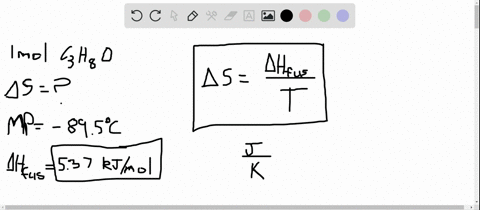Enroll in one of our FREE online STEM summer camps. Space is limited so join now!View Summer Courses### Calculate the change in entropy that occurs in th…

02:19
LP

Need more help? Fill out this quick form to get professional live tutoring.

Get live tutoring
Problem 30

Two systems, each composed of three particles represented by circles, have 30 $\mathrm{J}$ of total energy. How many energetically equivalent ways can you distribute the particles in each system?
Which system has greater entropy?

There are a total of $1 !=1$ energetically equivalent ways of distributing the
particles in System A. There are a total of $3 !=6$ energetically equivalent
ways of distributing the particles in System B. Therefore, System B has the
greater entropy.

## Discussion

You must be signed in to discuss.

## Video Transcript

So there's two systems will call it system, eh? Assistant D and the each are composed of three particles, and they have to have have a total of 30 jewels of energy total. So we'll call. This wax is energy. Now, the way you can arrange System A's, you can have all three particles be at 10 joules of energy. And there's not even though you can change these where they are at the same spot. It's not actual different microbes state. So those stay the same system being you could do something such as this. Go one above the 10 jewels and two below potentials. So those two will be a fab Jules. So it's you jumped five is 10 and then one times twenties 20. So you have 30 total still, but now you can split up different ways. So we call this particle one article too. EMP, Article three. You can arrange this another way. You could have particle two be here. Article one be here and three still be here. You can also do something like this where theory is at the top and one and two are at the five jewels energy level. It says three different ways. You can arrange this system that are energetically equipment versus one. So you would say system, he has greater entropy in this case.